# 动转静两大升级！一键转静成功率领先，重点模型训练提速 18%+

• 2023-03-07
北京
• 本文字数：3447 字

阅读完需：约 11 分钟

• 动转静成功率明显提升，一键转换成功率达到 92.1%。

• 动转静训练加速效果明显，重点模型训练可提速 18%+。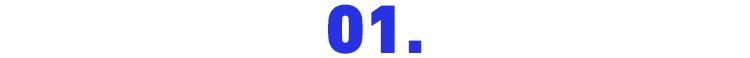JIT 式动态执行API 动静行为统一

Reduce 系列的 paddle.mean/sum/max/min API 的参数 axis ，新增支持为 Tensor 类型，动态可变。

Einsum 升级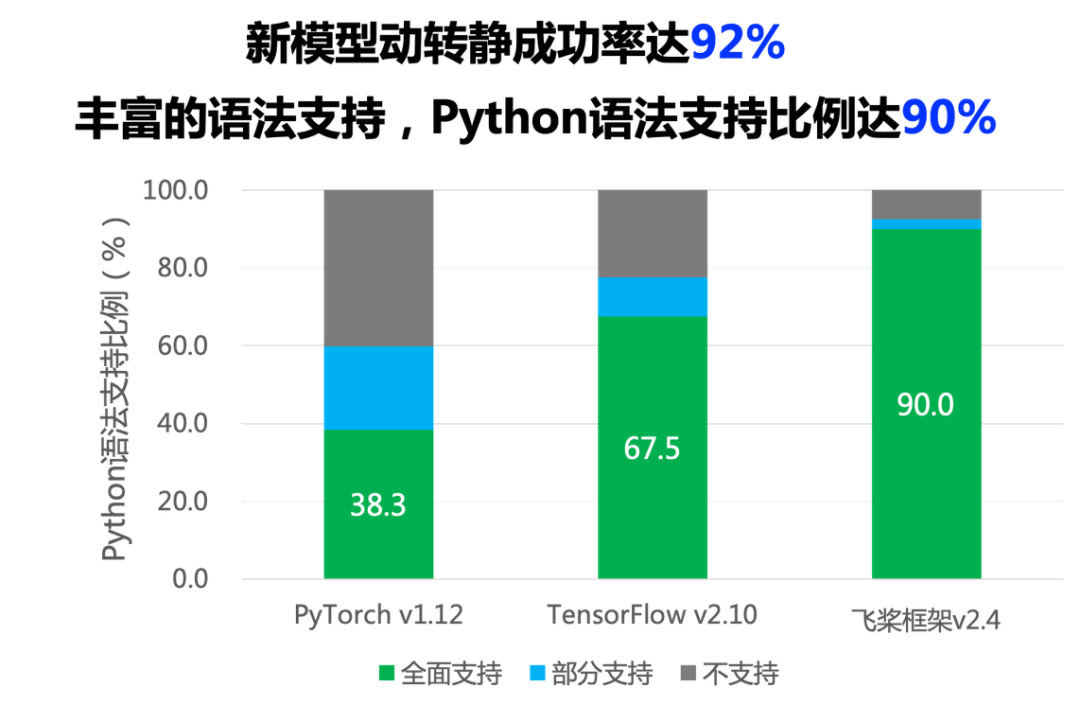import paddleclass SimpleNet(paddle.nn.Layer):    def __init__(self):        super(SimpleNet, self).__init__()        self.linear = paddle.nn.Linear(10, 3)    @paddle.jit.to_static   # step 1： 添加装饰器    def forward(self, x):        out = self.linear(x)        out = out + 1        return outnet = SimpleNet()train(net)  # 此处略去了训练过程# step 2: 切换到 eval() 模式net.eval()# step 3: 调用 jit.save 接口paddle.jit.save(net, path='./simple_net')

simple_net.pdiparams        // 存放模型中所有的权重数据simple_net.pdmodel          // 存放模型的网络结构simple_net.pdiparams.info   // 存放额外的其他信息

• 添加装饰器

• 切换 eval 模式

• 调用 save 接口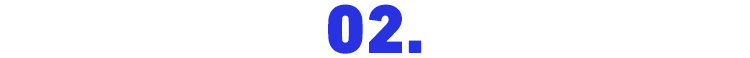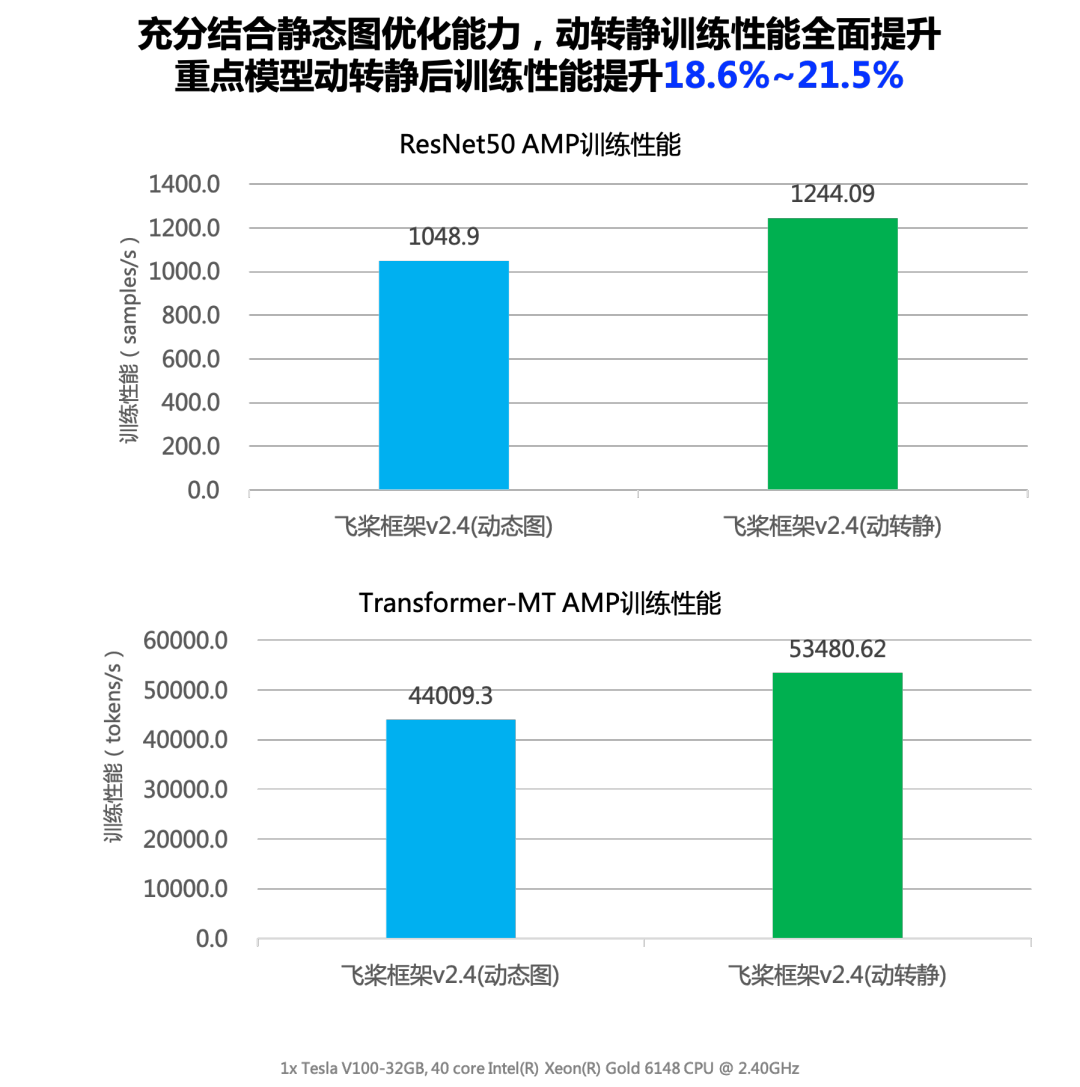• PU 利用率较低（可通过 watch -n 1 nvidia-smi 命令查看）。

• 常见于 NLP 领域或 AMP/FP16 任务。

• 训练性能瓶颈点主要是 Host 端调度开销。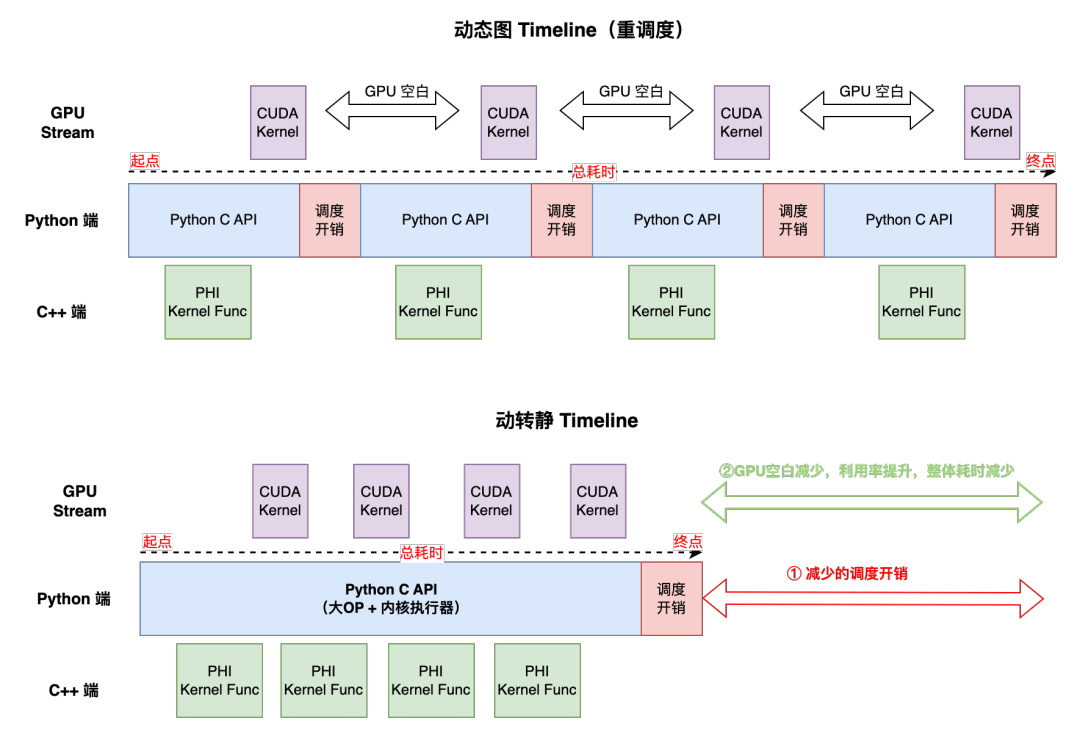• 一个 Batch 的训练耗时取决于 Host 端总耗时。

• 动态图每个 Python API 在运行时，都会产生一次 Python 和 C++交互，会产生较大的调度开销。

• 动转静之后，整体上切分为执行前向和反向的两个 Python C API，故减少了很多个 API 间的调度开销。

• 动转静内核执行器也经过了极致的优化（如 Instruction 缓存等），Kernel launch 效率也会比纯动态图模式要高。

import paddleclass SimpleNet(paddle.nn.Layer):    def __init__(self):        super(SimpleNet, self).__init__()        self.linear = paddle.nn.Linear(10, 3)    @paddle.jit.to_static   # 仅需一行代码    def forward(self, x):        out = self.linear(x)        out = out + 1        return out# create networknet = SimpleNet()adam = opt.Adam(learning_rate=0.001, parameters=net.parameters())for batch_id, x in enumerate(data_loader()):    out = net(x)    loss = paddle.mean(out)    loss.backward()    opt.step()    opt.clear_grad()• GPU 利用率波动比较大（可通过 watch -n 1 nvidia-smi 命令查看）。

• 训练性能瓶颈点同时受局部调度和局部 Kernel 计算效率影响。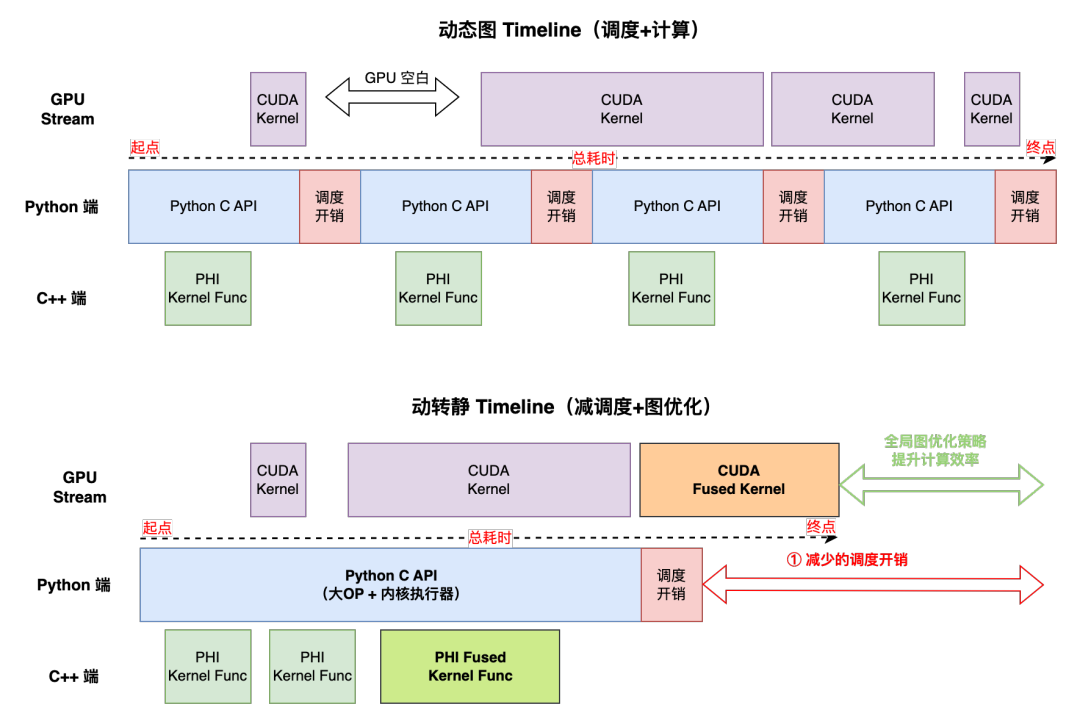• 一个 Batch 的训练耗时取决于 Max(Host 端，GPU 端)。

• 动转静降低了 Python C API 调度开销，收益点大多在 Batch 前半部分，后半部分可能会被 overlap，调度方面的收益会打折扣。

• 动转静可借助全局图优化技术，通过算子融合等技术提升模型训练的吞吐。

import numpy as npimport paddleimport paddle.nn as nndef get_build_strategy():    build_strategy = paddle.static.BuildStrategy()    # 算子融合策略    build_strategy.fuse_elewise_add_act_ops = True    # 梯度 addto 策略    build_strategy.enable_addto = True    os.environ['FLAGS_max_inplace_grad_add'] = "8"    return build_strategyclass ResNet(paddle.nn.Layer):    # 此处省略了模型定义    @to_static(build_strategy=get_build_strategy()) # 方式一    def forward(self, image):        # 省略前向代码# create networknet = ResNet()# 借助 build_strategy 参数自定义开启「全局图优化」策略net = paddle.jit.to_static(net, build_strategy=get_build_strategy()) # 方式二adam = opt.Adam(learning_rate=0.001, parameters=net.parameters())for batch_id, image in enumerate(data_loader()):    out = layer(image)    loss = paddle.mean(out)    loss.backward()    opt.step()    opt.clear_grad()